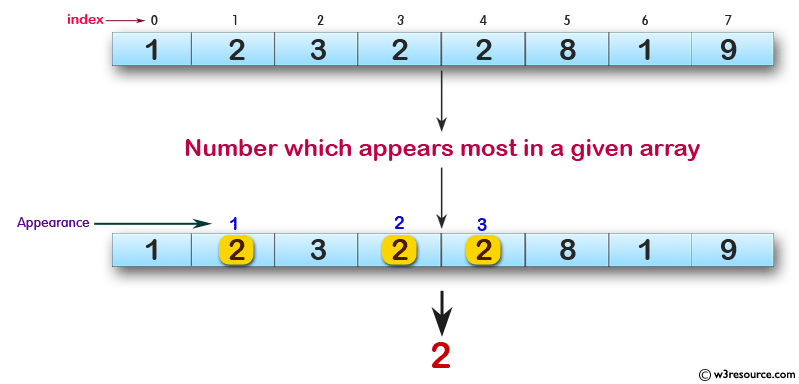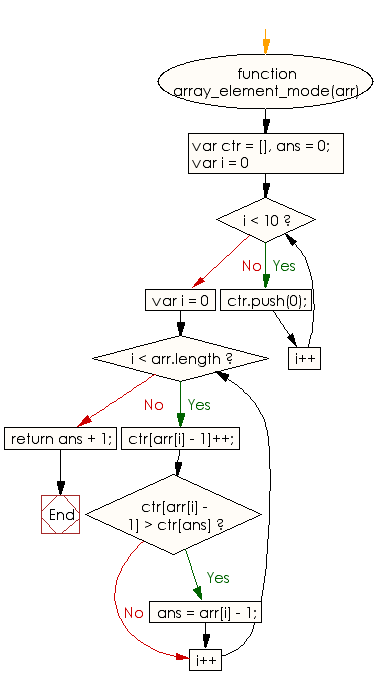# JavaScript: Find the number which appears most in a given array of integers

## JavaScript Basic: Exercise-94 with Solution

Write a JavaScript program to find the number which appears most in a given array of integers.

Pictorial Presentation:Sample Solution:

HTML Code:

``````<!DOCTYPE html>
<html>
<meta charset="utf-8">
<meta name="viewport" content="width=device-width">
<title>Find the number which appears most in a given array of integers</title>
<body>

</body>
</html>
```
```

JavaScript Code:

``````function array_element_mode(arr) {
var ctr = [],
ans = 0;

for(var i = 0; i < 10; i++) {
ctr.push(0);
}
for(var i = 0; i < arr.length; i++) {
ctr[arr[i] - 1]++;
if(ctr[arr[i] - 1] > ctr[ans]) {
ans = arr[i] - 1;
}
}
return ans + 1;
}
console.log(array_element_mode([1, 2, 3, 2, 2, 8, 1, 9]))
```
```

Sample Output:

```2
```

Flowchart:ES6 Version:

``````function array_element_mode(arr) {
const ctr = [];
let ans = 0;

for(var i = 0; i < 10; i++) {
ctr.push(0);
}
for(var i = 0; i < arr.length; i++) {
ctr[arr[i] - 1]++;
if(ctr[arr[i] - 1] > ctr[ans]) {
ans = arr[i] - 1;
}
}
return ans + 1;
}
console.log(array_element_mode([1, 2, 3, 2, 2, 8, 1, 9]))
``````

Live Demo:

See the Pen javascript-basic-exercise-94 by w3resource (@w3resource) on CodePen.

What is the difficulty level of this exercise?

Test your Programming skills with w3resource's quiz.

﻿

## JavaScript: Tips of the Day

Checks if a string is an anagram of another string (case-insensitive, ignores spaces, punctuation and special characters)

Example:

```const isAnagram = (str1, str2) => {
const normalize = str =>
str
.toLowerCase()
.replace(/[^a-z0-9]/gi, '')
.split('')
.sort()
.join('');
return normalize(str1) === normalize(str2);
};
console.log(isAnagram('iceman', 'cinema')); // true
```

Output:

```true
```